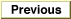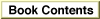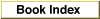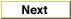# Legacy DocumentImportant: The information in this document is obsolete and should not be used for new development.

Inside Macintosh: QuickDraw GX Environment and Utilities /
Chapter 8 - QuickDraw GX Mathematics / QuickDraw GX Mathematics Reference
Mathematical Functions / Fixed-Point Operations

### FractSquareRoot

You can use the `FractSquareRoot` function to calculate the square root of a `fract` number.

```fract FractSquareRoot (fract source);
```
`source`
The number for which the square root is required.
function result
The square root of the `fract number.`
##### DESCRIPTION
The `FractSquareRoot` function returns the square root of the `fract` number specified by the `source` parameter. The number is interpreted as unsigned and in the range 0 through 4 - (2-30). This means that bit 31 has a weight of 2, instead of -2. The result is an unsigned number in the range of 0 through 2.